# Time value 2.27 points Kilgore Natural Gas has a \$1,000 par value bond outstanding that pays 13 percent annual inte...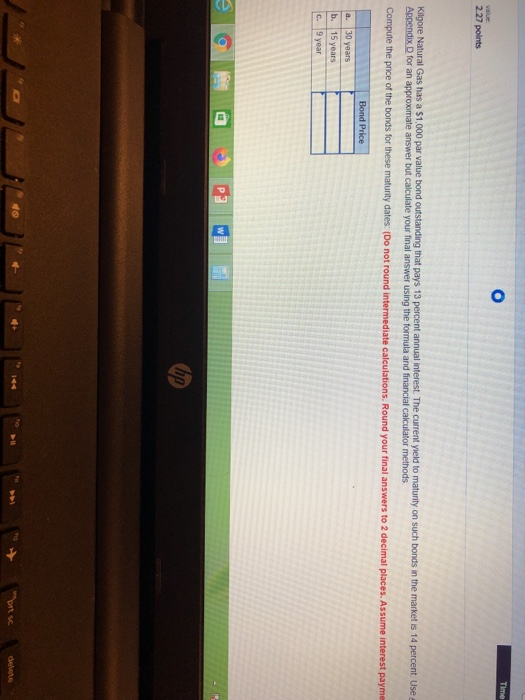Time value 2.27 points Kilgore Natural Gas has a \$1,000 par value bond outstanding that pays 13 percent annual interest. The current yield to maturity on such bonds in the market is 14 percent. Use a Appendix D for an approximate answer but calculate your final answer using the formula and financial calculator methods Compute the price of the bonds for these maturity dates: (Do not round intermediate calculations. Round your final answers to 2 decimal places. Assume interest payme Bond Price 30 years a. b. 15 years C 9 year hp prt sc delete 144

A: Using financial calculator
Input: FV= 1000

N=30, PMT=13%*1000=130

I/Y=14, Solve for PV as -929.97

Price of bond = \$929.97

B:

Using financial calculator
Input: FV= 1000

N=15, PMT=13%*1000=130

I/Y=14, Solve for PV as -938.58

Price of bond = \$938.58

C:

Using financial calculator
Input: FV= 1000

N=9, PMT=13%*1000=130

I/Y=14, Solve for PV as -950.54

Price of bond = \$950.54

##### Add Answer of: Time value 2.27 points Kilgore Natural Gas has a \$1,000 par value bond outstanding that pays 13 percent annual inte...
Similar Homework Help Questions
• ### Kilgore Natural Gas has a \$1,000 par value bond outstanding that pays 18 percent annual interest....

Kilgore Natural Gas has a \$1,000 par value bond outstanding that pays 18 percent annual interest. The current yield to maturity on such bonds in the market is 12 percent. Use Appendix B and Appendix D for an approximate answer but calculate your final answer using the formula and financial calculator methods. Compute the price of the bonds for these maturity dates: (Do not round intermediate calculations. Round your final answers to 2 decimal places. Assume interest payments are annual.)...

• ### Essex Biochemical Co. has a \$1,000 par value bond outstanding that pays 12 percent annual interest....

Essex Biochemical Co. has a \$1,000 par value bond outstanding that pays 12 percent annual interest. The current yield to maturity on such bonds in the market is 10 percent. Use Appendix B and Appendix D for an approximate answer but calculate your final answer using the formula and financial calculator methods. Compute the price of the bonds for the maturity dates: (Do not round intermediate calculations. Round your final answer to 2 decimal places. Assume interest payments are annual.)...

• ### Applied Software has a \$1,000 par value bond outstanding that pays 13 percent interest with annual...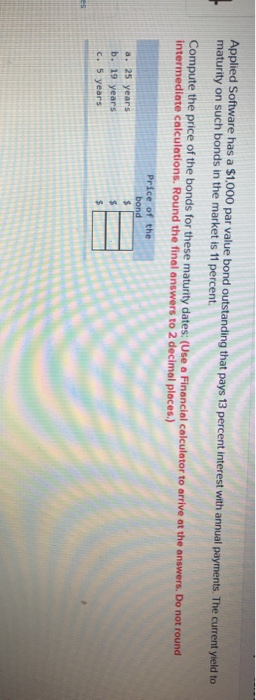Applied Software has a \$1,000 par value bond outstanding that pays 13 percent interest with annual payments. The current yield to maturity on such bonds in the market is 11 percent Compute the price of the bonds for these maturity dates: (Use a Financial calculator to arrive at the answers. Do not round intermediate calculations. Round the final answers to 2 decimal places.) Price of the bond \$ a. 25 years b. 19 years \$ c. 5 years \$

• ### BP has a \$1,000 par value bond outstanding that pays 12 percent annual interest. The current...

BP has a \$1,000 par value bond outstanding that pays 12 percent annual interest. The current yield to maturity on such bonds in the market is 10 percent. Compute the price of the bonds for the following maturity dates. Show all your work IN EXCEL for full credit. a. 30 years b. 10 years c. 5 years

• ### Heather Smith is considering a bond investment in Locklear Airlines. The \$1,000 par value bonds have...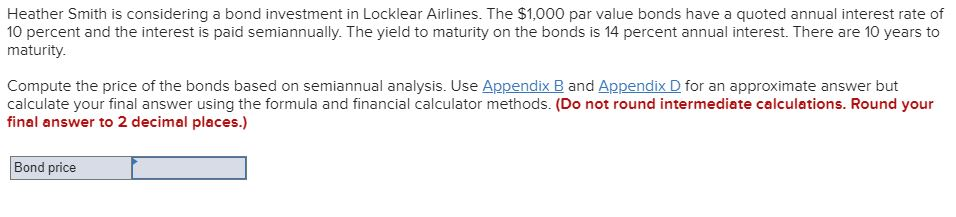Heather Smith is considering a bond investment in Locklear Airlines. The \$1,000 par value bonds have a quoted annual interest rate of 10 percent and the interest is paid semiannually. The yield to maturity on the bonds is 14 percent annual interest. There are 10 years to maturity. Compute the price of the bonds based on semiannual analysis. Use Appendix B and Appendix D for an approximate answer but calculate your final answer using the formula and financial calculator methods....

• ### Heather Smith is considering a bond investment in Locklear Airlines. The \$1,000 par value bonds have...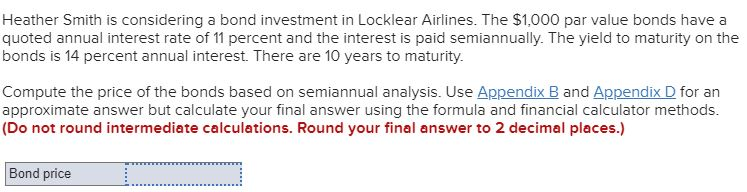Heather Smith is considering a bond investment in Locklear Airlines. The \$1,000 par value bonds have a quoted annual interest rate of 11 percent and the interest is paid semiannually. The yield to maturity on the bonds is 14 percent annual interest. There are 10 years to maturity. Compute the price of the bonds based on semiannual analysis. Use Appendix B and Appendix D for an approximate answer but calculate your final answer using the formula and financial calculator methods....

• ### The Lone Star Company has \$1,000 par value bonds outstanding at 9 percent interest. The bonds...

The Lone Star Company has \$1,000 par value bonds outstanding at 9 percent interest. The bonds will mature in 20 years. Use Appendix B and Appendix D for an approximate answer but calculate your final answer using the formula and financial calculator methods. Compute the current price of the bonds if the present yield to maturity is. (Do not round intermediate calculations. Round your final answers to 2 decimal places. Assume interest payments are annual.) c. 13 percent

• ### Heather Smith is considering a bond investment in Locklear Airines. The \$1,000 par value bonds have...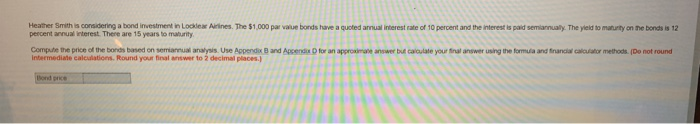Heather Smith is considering a bond investment in Locklear Airines. The \$1,000 par value bonds have a quoted annual interest rate of 10 percent and the interest is paid semiannualy. The yield to maturty on the bonds is 12 percent annual interest. There are 15 years to maturity Compute the price of the bonds based on semiannual analysis. Use Appendix B and Appendix D for an approximate answer but caloulate your final answer using the formula and financial calculator methods....

• ### The Lone Star Company has \$1.000 par value bonds outstanding at 10 percent interest. The bonds...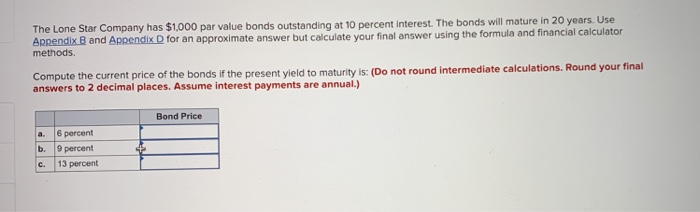The Lone Star Company has \$1.000 par value bonds outstanding at 10 percent interest. The bonds will mature in 20 years Use Appendix B and Appendix D for an approximate answer but calculate your final answer us methods ing the formula and financial calculator Compute the current price of the bonds if the present yield to maturity is: (Do not round intermediate calculations. answers to 2 decimal places. Assume interest payments are annual.) Bond Price a. 6 percent b. 9...

• ### Standard Olive Company of California has a \$1,000 par value convertible bond outstanding with a coupon...

Standard Olive Company of California has a \$1,000 par value convertible bond outstanding with a coupon rate of 8 percent and a maturity date of 20 years. It is rated Aa, and competitive, nonconvertible bonds of the same risk class carry a 22 percent yield. The conversion ratio is 20. Currently the common stock is selling for \$30 per share on the New York Stock Exchange. a. What is the conversion price? (Round your answer to 2 decimal places.) Conversion...

Need Online Homework Help?